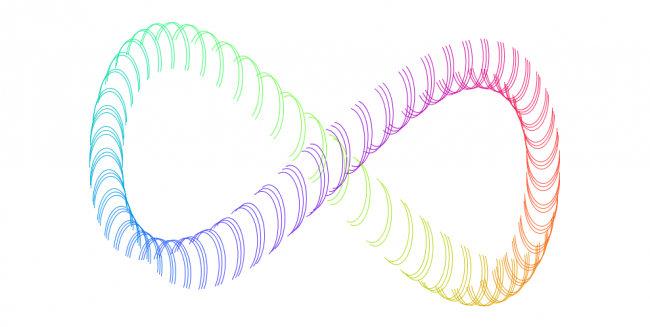#### Howdy, Stranger!

We are about to switch to a new forum software. Until then we have removed the registration on this forum.

# Figuring out Values for Arc Rotation OscillationAs you can see in this picture the arcs rotation, or start and stop values, change using the code as follows:

``````arcAngle1 = Math.sin(freq*i) * arcAngle1Span + arcAngle1Start;
arcAngle2 = Math.sin(freq*i) * arcAngle2Span + arcAngle2Start;`
``````

where:

``````arcAngle1Start = -HALF_PI;
arcAngle1Span = HALF_PI;
arcAngle2Start = HALF_PI;
arcAngle2Span= HALF_PI;
``````

I am trying to figure out the values needed for it to sync so that the middle of the arc is always pointing in the so called direction of travel. It is possible that something else is wrong entirely and I'm thinking using the wrong method so here is the entire code for that reason: https://pastebin.com/nHFmHtYz

Thank you all in advance for any help.

Tagged:

## Answers

• the middle of the arc is always pointing in the so called direction of travel.

I cannot see how the middle of the arc points in the direction of travel. If we start with a circle in the XY plane and a direction vector at the center of this circle and pointing along Z, how would you draw an arc pointing in that direction?

Kf

• 1) Why are you using doubles for position, angle and colour information? It will not make any difference to the displayed output.

2) If you insist on keeping the doubles then should should be aware that
`Float.parseFloat(new Double(colR).toString())`
is NOT the way to convert a double to a float. It is extremely innefficient and should be replaced with
`(float) colR`
which does the same thing.

I have modified your program (code below) to remove the doubles. You will find that it is much faster.

Regarding direction of travel.

In your code each arc is part of the circumference of an ellipse . It is impossible to align the arcs to the direction of travel by simply changing the start and end angles of the arc. The solution is to calculate the angle of travel and then rotate the arc before it is drawn (NOTE every arc will have the same start and end angle).

Additional comment:
If each arc was part of the circumference of a circle then it would be possible to use the start and end angles

``````float colUpdate = 0.0;
float len = PI;

float freq = .1;

//Color Variables
float colR = 0.0;
float colG = 0.0;
float colB = 0.0;

//Color Oscillation Variables
int colorStart = 128;
int colorSpan = 127;

//Arc Position Variables
float xPos = 0.0;
float yPos = 0.0;

//Arc Position Oscillation Variables
int widthStart = 0;
int widthSpan = 0;
int heightStart = 0;
int heightSpan = 0;

//Arc Rotation Variables
float arcAngle1 = 0.0;
float arcAngle2 = 0.0;

//Arc Rotation Oscillation variables
float arcAngle1Start = 0.0;
float arcAngle1Span = 0.0;
float arcAngle2Start = 0.0;
float arcAngle2Span = 0.0;

void setup() {
size(1000, 500);
frameRate(10);
background(255, 255, 255);
}

void draw() {
//Misc
len += 1;
noFill();

//Setting Values for Arc Position Oscillation
widthStart = width/2;
widthSpan = width/3;
heightStart = height/2;
heightSpan = height/3;

//Setting Values for Arc Rotation Oscillation
arcAngle1Start = -HALF_PI;
arcAngle1Span = HALF_PI;
arcAngle2Start = HALF_PI;
arcAngle2Span= HALF_PI;

//Sine Oscillation for Color and Arc Position
for (int i = 0; i < len; ++i)
{
colR = (float) Math.sin(freq*i + 0) * colorSpan + colorStart;
colG = (float) Math.sin(freq*i + 2) * colorSpan + colorStart;
colB = (float) Math.sin(freq*i + 4) * colorSpan + colorStart;

xPos = (float) Math.sin(freq*i) * widthSpan + widthStart;
yPos = (float) Math.sin((2*freq)*i) * heightSpan + heightStart;

arcAngle1 = (float) Math.sin(freq*i) * arcAngle1Span + arcAngle1Start;
arcAngle2 = (float) Math.sin(freq*i) * arcAngle2Span + arcAngle2Start;
}

//Choosing Color of Arc Using the Color Doubles Converted to Floats
stroke(colR, colG, colB);

//Drawing the Arc Using the Same Float Conversion Method
arc(xPos, yPos, 50, 100, arcAngle1, arcAngle2);
}
``````
• Thanks a lot for the feedback! You can probably tell that I'm new to processing by the way i am writing my code and as such i really appreciate the response.

Sign In or Register to comment.